NEET  >  Refraction at Spherical Surface & Lenses

# Refraction at Spherical Surface & Lenses - Notes | Study Physics Class 12 - NEET

 Table of contentsRefraction at a Spherical SurfaceLensTypes of Lens1 Crore+ students have signed up on EduRev. Have you?

Refraction at a Spherical Surface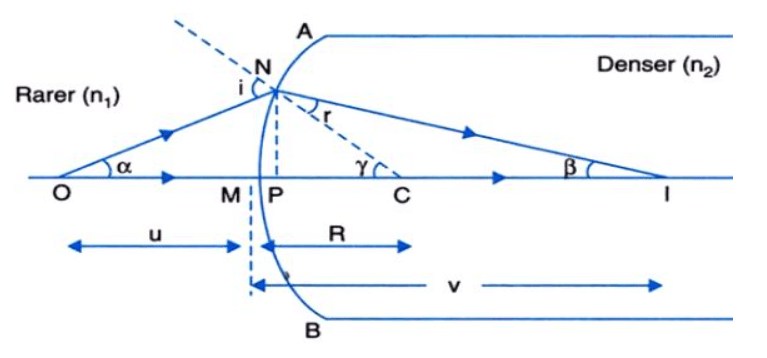• Let us now see the refraction of light at the spherical surface. Now, the change in direction or bending of a light wave passing from one transparent medium to another caused by the change in wave’s speed is the Refraction.
• Suppose the above figure is a spherical surface. There is one medium with refractive index n1 and the second medium with refractive index n2.
• There is an object O and a ray of light from the object O is incident on the spherical mirror. Since it is moving from a rarer medium to a denser medium, the ray bends towards the normal.
• An image is formed and the radius of curvature of a spherical surface is R with the center C of the spherical surface.

Now as we know that:

• n1 is the refractive index of a medium from which rays are incident.
• n2 is the refractive index of another medium

We get:
► tanα = MN / OM
► tanγ = MN / MC
► tanβ = MN / MI
Now, for Δ NOC, i is the exterior angle.
i = ∠ NOM + ∠ NCM
i= MN / OM + MN / MC   ...(1)
Similarly,
r =  MN / MC – MN / MI  ...(2)
Now by using Snell’s law we get:
n1 sin i = n2sin r
Substituting i and r from Eq. (1) and (2), we get
n/ OM + n/ MI = (n− n1) / MC
As, OM = -u, MI = +v, MC = +R
Hence, the equation becomes: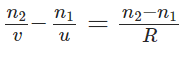Lens

A lens is a uniform transparent medium bounded between two spherical or one spherical and one plane surface.
Here are some terms related to Lens:

• Centre of Curvature: The centre of the actual glass sphere, of which your lens forms a part
• Principal Axis: When two spheres are part of your lens, it is the imaginary line joining the centres of curvatures of both spheres.
• Principal Focus: It is point on the principal axis, where light rays parallel to principal axis meet in case of a convex lens (or appear to meet after extrapolation in case of a concave lens).
• Optical Centre: It is a point within the lens where the diameter of the lens and the principal axis meet
• Focal Length: The distance between the focus and the optical centre

Types of Lens

1. Convex Lens
A lens that is thinner at the edges and thicker at the middle is called a convex or converging lens. A convex lens is also referred to as a converging lens since it “converges” light rays that are incident on it.
2. Concave Lens
A lens which is thicker at edges and thinner at middle, is called a concave or diverging lens. A concave lens is also referred to as a diverging lens since it “diverges” light rays that are incident on it.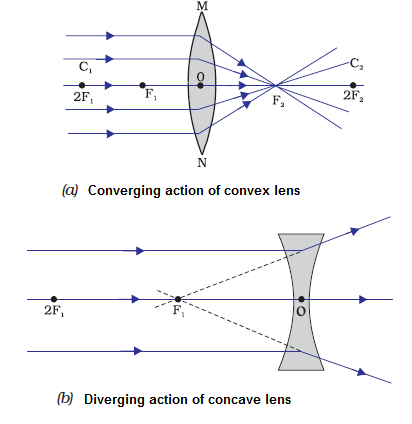Convex and Concave Lens

➢ Lens Formula
1/f = 1/v – 1/u
where, f = focal length of the lens, U = distance of object, U = distance of image.
Lens Maker’s formula
1/f=(μ – 1) (1/R1 – 1/R2)
where, μ = refractive index of the material of the lens and R1 and R2 are radii of curvature of the lens.
➢ Power of a Lens
The reciprocal of the focal length of a lens, when it is measured in metre, is called power of a lens.
Power of a lens, (P)= 1/f(metre)
Its unit is dioptre (D).
The power of a convex (converging) lens is positive and for a concave (diverging) lens it is negative.

➢ Focal Length of a Lens Combination
(i) When lenses are in contact 1/F – 1/f1 + 1/f2
Power of the combination P = P1 + P2

(ii) When lenses are separated by a distance d
1/F = 1/f1 + 1/f2 – d/f1f1
Power of the combination:
P = P1 + P2 – dP1P2

➢ Linear Magnification
m = I/O = v/u
For a small sized object placed linearly along the principal axis, its axial (longitudinal) magnification is given by
Axial magnification = – dv/du = (v/u)2
= (f / f+u)2 = (f - v/f)2

➢ Focal Length of a Convex Lens by Displacement Method
Focal length of the convex lens f = (a2 – d2) / 4a
where, a = distance between the image pin and object pin and
d = distance between two positions of lens.
The distance between the two pins should be greater than four times the focal length of the convex lens, i.e., a > 4f.
Height of the object O = √I1I2

➢ Cutting of a Lens

• If a symmetrical convex lens of focal length f is cut into two parts along its optic axis, then focal length of each part (a plane convex lens) is 2f. However, if the two parts are joined as shown in figure, the focal length of combination is again f.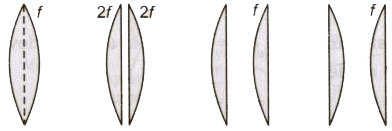• If a symmetrical convex lens of focal length f is cut into two parts along the principal axis, then focal length of each part remains unchanged as f. If these two parts are joined with curved ends an one side, focal length of the combination is f/2. But on joining two 2 parts in opposite sense the net focal length becomes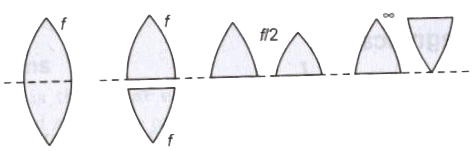The document Refraction at Spherical Surface & Lenses - Notes | Study Physics Class 12 - NEET is a part of the NEET Course Physics Class 12.
All you need of NEET at this link: NEET

## Physics Class 12

157 videos|452 docs|213 tests

## Physics Class 12

157 videos|452 docs|213 tests

### How to Prepare for NEET

Read our guide to prepare for NEET which is created by Toppers & the best Teachers

Track your progress, build streaks, highlight & save important lessons and more!

,

,

,

,

,

,

,

,

,

,

,

,

,

,

,

,

,

,

,

,

,

;